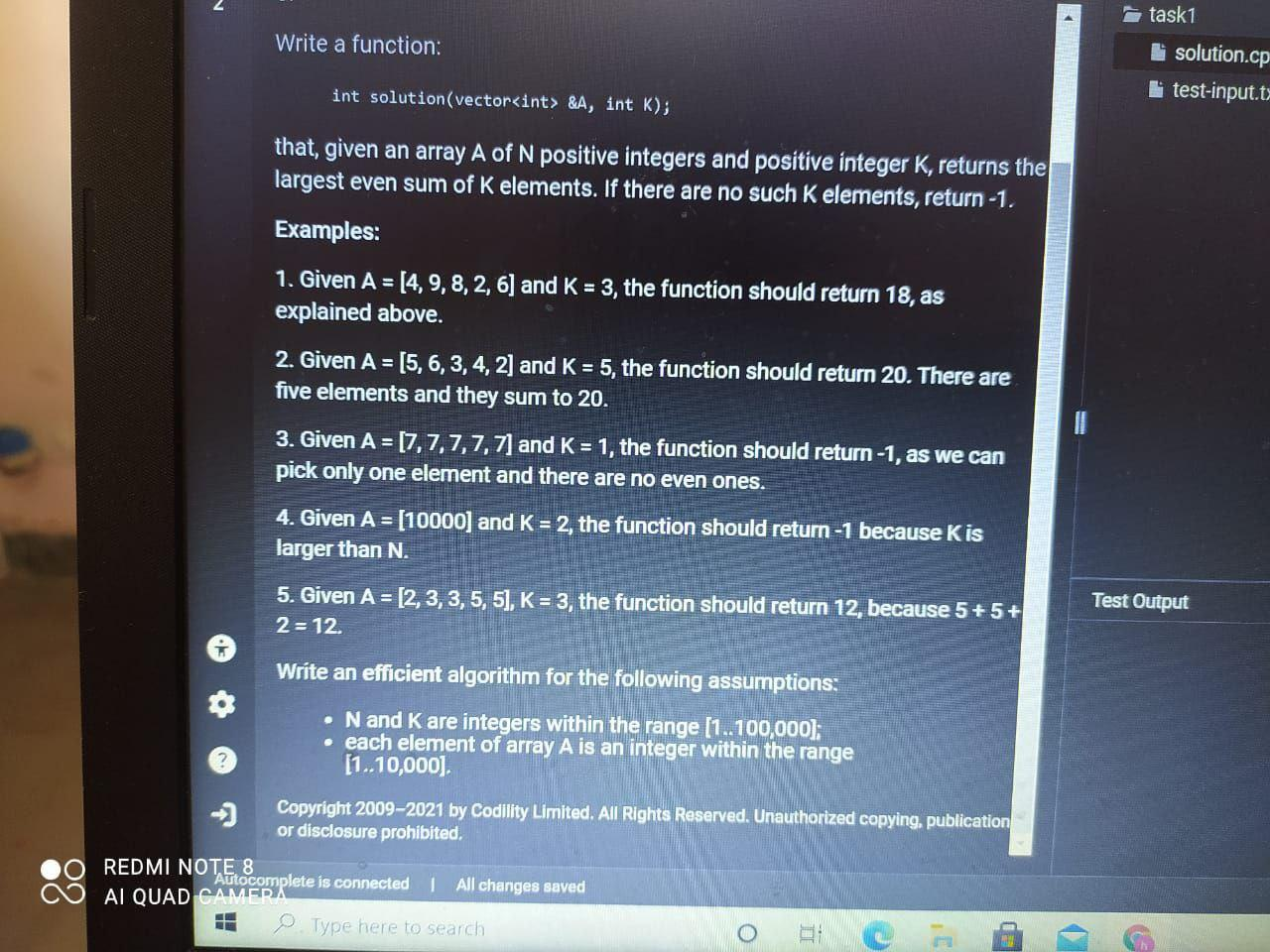# Question task1 Write a function: solution.cp test-input.t- int solution (vector<int> &A, int k); that, given an array A of N positive integers and positive integer K, returns the largest even sum of K elements. If there are no such k elements, return -1. Examples: 1. Given A = [4, 9, 8, 2, 6] and K = 3, the function should return 18, as explained above. 2. Given A = [5, 6, 3, 4, 2) and K = 5, the function should return 20. There are five elements and they sum to 20. 3. Given A = [7,7,7,7,7] and K = 1, the function should return -1, as we can pick only one element and there are no even ones. 4. Given A = [10000) and K = 2, the function should return -1 because K is larger than N. 5. Given A = [2,3,3,5, 5), K = 3, the function should return 12, because 5+5+ 2 = 12. Test Output Write an efficient algorithm for the following assumptions: • N and K are integers within the range [1..100,000); • each element of array A is an integer within the range [1..10,000). -- Copyright 2009-2021 by Codility Limited. All Rights Reserved. Unauthorized copying, publication or disclosure prohibited. REDMI NOTE 8 Autocomplete is connected 1 All changes saved AI QUAD CAMERA o Type here to searchEM06ZV The Asker · Computer ScienceTranscribed Image Text: task1 Write a function: solution.cp test-input.t- int solution (vector &A, int k); that, given an array A of N positive integers and positive integer K, returns the largest even sum of K elements. If there are no such k elements, return -1. Examples: 1. Given A = [4, 9, 8, 2, 6] and K = 3, the function should return 18, as explained above. 2. Given A = [5, 6, 3, 4, 2) and K = 5, the function should return 20. There are five elements and they sum to 20. 3. Given A = [7,7,7,7,7] and K = 1, the function should return -1, as we can pick only one element and there are no even ones. 4. Given A = [10000) and K = 2, the function should return -1 because K is larger than N. 5. Given A = [2,3,3,5, 5), K = 3, the function should return 12, because 5+5+ 2 = 12. Test Output Write an efficient algorithm for the following assumptions: • N and K are integers within the range [1..100,000); • each element of array A is an integer within the range [1..10,000). -- Copyright 2009-2021 by Codility Limited. All Rights Reserved. Unauthorized copying, publication or disclosure prohibited. REDMI NOTE 8 Autocomplete is connected 1 All changes saved AI QUAD CAMERA o Type here to search
More
Transcribed Image Text: task1 Write a function: solution.cp test-input.t- int solution (vector &A, int k); that, given an array A of N positive integers and positive integer K, returns the largest even sum of K elements. If there are no such k elements, return -1. Examples: 1. Given A = [4, 9, 8, 2, 6] and K = 3, the function should return 18, as explained above. 2. Given A = [5, 6, 3, 4, 2) and K = 5, the function should return 20. There are five elements and they sum to 20. 3. Given A = [7,7,7,7,7] and K = 1, the function should return -1, as we can pick only one element and there are no even ones. 4. Given A = [10000) and K = 2, the function should return -1 because K is larger than N. 5. Given A = [2,3,3,5, 5), K = 3, the function should return 12, because 5+5+ 2 = 12. Test Output Write an efficient algorithm for the following assumptions: • N and K are integers within the range [1..100,000); • each element of array A is an integer within the range [1..10,000). -- Copyright 2009-2021 by Codility Limited. All Rights Reserved. Unauthorized copying, publication or disclosure prohibited. REDMI NOTE 8 Autocomplete is connected 1 All changes saved AI QUAD CAMERA o Type here to search